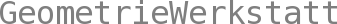Integrable Systems
SS 2014

Prof Dr Christoph Bohle
Assistant: Dr Aaron Gerding (email)

Lecture
Tuesday/Thursday, 12-14, N14

Practice Session
Monday, 14-16, S8 (5th floor)

Exercise Sets

Overview of the Lecture

Taken in full generality, the term “integrable systems” refers to a vast swath of terrain stretching between various often far removed frontiers in mathematics and physics that can perhaps only be characterized by the presence of differential (or difference) equations - the “systems” - possessing an exceptional degree of symmetry - and therefore considered “integrable” in some sense. But in spite their expansive range, such systems are exceedingly rare creatures, and live among the general population much as the Platonic solids do among the set of all polyhedrons.

The focus of this lecture will be on a particular region of this terrain known as “soliton theory” which studies very special non-linear PDE’s (and their discrete analogues) describing wave phenomena in classical and quantum physics where the solutions, while not allowing superposition as in the linear theory, nevertheless behave much more like “building-blocks” than for a generic wave equation. Here, integrability has a clearer meaning: the solutions and their interactions have explicit expressions in terms of parameters for the (generally infinite dimensional) group of symmetries for the system. To derive such expressions we will follow the highly conceptual approach of the Kyoto school initiated by Sato which encodes the symmetry group as transformations of an infinite dimensional Grassmannian, and which uses the formalism of the quantum theory of elementary particles in intriguing ways. We will then have a convenient language in which to investigate some surprising connections between those seemingly far removed frontiers, traversing in particular through interesting areas of classical and differential geometry.

As further motivation we quote Phillip Griffiths:
"One of the most remarkable achievements of mathematics of the latter half of the 20th century is the theory of solitons, which illustrates the underlying unity of the field. Solitons are nonlinear waves that exhibit extremely unexpected and interesting behavior. ...

This discovery provoked a rush of activity, which exhibited in the most beautiful way the unity of mathematics. It involved developments in computation, and in mathematical analysis, which is the traditional way to study differential equations. It turns out that one can understand the solutions to these differential equations through certain very elegant constructions in algebraic geometry. The solutions are also intimately related to representation theory, in that these equations turn out to have an infinite number of hidden symmetries. Finally, they relate back to problems in elementary geometry."

- Mathematics at the Turn of the Millennium, The American Mathematical Monthly, Vol. 107, No. 1 (Jan., 2000), pp. 1-14

Prerequisites
Students are expected to have completed Analysis IV and have a basic knowledge of manifolds (as provided by, e.g., Introduction to Manifolds or Mathematical Physics I).

Suggested Literature